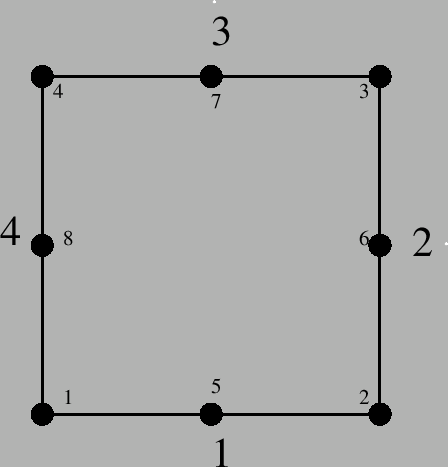### Eight-node plane stress element (CPS8 and CPS8R)

The eight node plane stress element is a general purpose plane stress element. It is actually a special case of shell element: the structure is assumed to have a symmetry plane parallel to the x-y plane and the loading only acts in-plane. In general, the z-coordinates are zero. Just like in the case of the shell element, the plane stress element is expanded into a C3D20 or C3D20R element. Figures 68 and 69 apply. From the above premises the following conclusions can be drawn:

• The displacement in z-direction of the midplane is zero. This condition is introduced in the form of SPC's. MPC's must not be defined in z-direction!
• The displacements perpendicular to the z-direction of nodes not in the midplane is identical to the displacements of the corresponding nodes in the midplane.
• The normal is by default (0,0,1)
• The thickness can vary. It can be defined in the same way as for the shell element, except that the *SOLID SECTION card is used instead of the *SHELL SECTION card.
• Different offsets do not make sense.
• Point loads are treated in a similar way as for shells.

The use of plane stress elements can also lead to knots, namely, if the thickness varies in a discontinuous way, or if plane stress elements are combined with other 1D or 2D elements such as axisymmetric elements. The connection with the plane stress elements, however, is modeled as a hinge.

Distributed loading in plane stress elements is different from shell distributed loading: for the plane stress element it is in-plane, for the shell element it is out-of-plane. Distributed loading in plane stress elements is defined on the *DLOAD card with the labels P1 up to P4. The number indicates the face as defined in Figure 71.If a plane stress element is connected to a structure consisting of 3D elements the motion of this structure in the out-of-plane direction (z-direction) is not restricted by its connection to the 2D elements. The user has to take care that any rigid body motion of the structure involving the z-direction is taken care of, if appropriate. This particularly applies to any springs connected to plane stress elements, look at test example spring4 for an illustration.

Notice that structures containing plane stress elements should be defined in the global x-y plane, i.e. z=0 for all nodes.